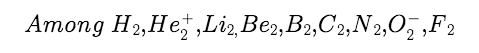# Find number of diamagnetic species?The number of diamagnetic species is

(Atomic numbers: H = 1, He = 2, Li = 3, Be = 4, B = 5, C = 6, N = 7, O = 8, F = 9)

Preeti Rai Chemistry 16 Dec, 2017 93 views